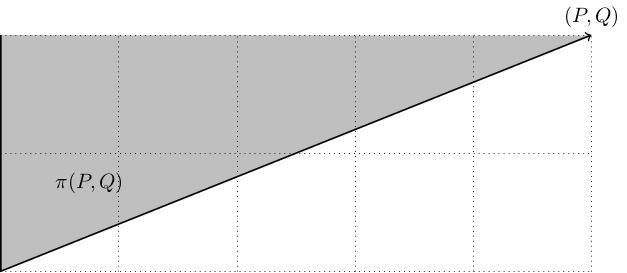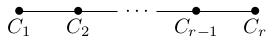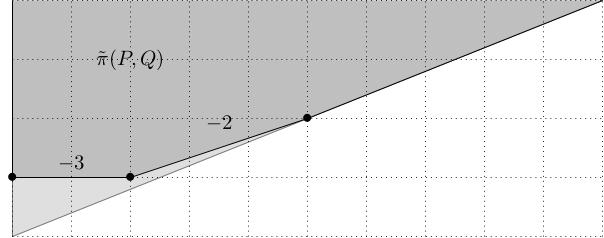# Cyclic quotient singularities

[2018-06-02 Sat]

I have been reading the paper "Flipping surfaces" by Hacking, Tevelev and Urzúa, which is a detailed study of a certain class of 3-fold flips. There is some background material about cyclic quotient surface singularities which I keep having to figure out from first principles whenever I return to this stuff and it would be helpful to have it written down somewhere. To that end, here is the first in a series of posts about this, covering the toric model for cyclic quotient singularities and the minimal resolution.

## Toric picture of cyclic quotient singularities

Given coprime positive integers $$P,Q$$, the cyclic quotient singularity of type $$\frac{1}{P}(1,Q)$$ is defined to be the quotient $$\mathbf{C}^2/\Gamma$$ where $$\Gamma$$ is the action of the group of $$P^{th}$$ roots of unity on $$\mathbf{C}^2$$ given by $$\mu\cdot(x,y)=(\mu x,\mu^Q y)$$.

The singularity $$\mathbf{C}^2/\Gamma$$ admits a Hamiltonian torus action generated by a moment map $$\mu\colon\mathbf{C}^2/\Gamma\to\mathbf{R}^2$$ whose image is the wedge $\pi(P,Q):=\{(x,y)\in\mathbf{R}^2\ :\ x\geq 0,\ Py\geq Qx\}.$The Hamiltonian functions $$H_1(x,y):=\frac{1}{2}|x|^2$$ and $$H_2(x,y):=\frac{1}{2}|y|^2$$ on $$\mathbf{C}^2/\Gamma_{P,Q}$$ Poisson-commute and generate an $$\mathbf{R}^2$$-action whose period lattice is the set of points $(\phi_1,\phi_2)=\left(2\pi\left(\frac{k}{P}+\ell\right), 2\pi\left(\frac{kQ}{P}+m\right)\right),\quad k,\ell,m\in\mathbf{Z}.$ (The period lattice for an $$\mathbf{R}^n$$-action is the set of points in $$\mathbf{R}^n$$ which act as the identity.) The Hamiltonians $$\frac{1}{P}(H_1+QH_2)$$ and $$H_2$$ therefore give us the standard period lattice $$2\pi\mathbf{Z}\oplus 2\pi\mathbf{Z}$$, therefore define an effective Hamiltonian torus action. The image of $$\mathbf{C}^2/\Gamma_{P,Q}$$ under $$\mu=\left(H_2,\frac{1}{P}(H_1+QH_2)\right)$$ is precisely the polygon $$\pi(P,Q)$$.

## Minimal resolution

The minimal resolution $$\pi\colon\tilde{X}\to X$$ of a cyclic quotient singularity $$X$$ of type $$\frac{1}{P}(1,Q)$$ has the following exceptional locus: it is a Hirzebruch-Jung chain of embedded spheres $$C_1,\ldots,C_r$$:with self-intersections $$C_i^2=-b_i$$, where $\frac{P}{Q}=[b_1,\ldots,b_r]:=b_1-\frac{1}{b_2-\frac{1}{\cdots-\frac{1}{b_r}}}.$ This is also toric; the moment polygon is obtained from $$\pi(P,Q)$$ by iteratively truncating the non-Delzant vertices (i.e. the vertices where the primitive integer vectors pointing along the outgoing edges do not form a basis for $$\mathbf{Z}^2$$) in much the way that blowing-up a smooth toric fixed point corresponds to truncating a Delzant vertex. The polygon $$\tilde{\pi}(P,Q)$$ is therefore a subset of $$\pi(P,Q)$$; below we see the minimal resolution for $$\frac{1}{5}(1,2)$$ (with $$b_1=3$$, $$b_2=2$$ and $$\frac{P}{Q}=\frac{5}{2}=3-\frac{1}{2}$$.From this toric picture we can easily verify that the self-intersections of the exceptional curves in the minimal resolution are the coefficients of the continued fraction of $$P/Q$$:
• The first truncation should be made horizontally: this is the obvious way to ensure that the left-most vertex is Delzant.
• The new right-hand vertex now has outgoing edge vectors $$(-1,0)$$ and $$(P,Q)$$; if we write $$P=b_1Q-R_1$$ for some $$0\leq R_1\leq Q-1$$ then the matrix $$\left(\begin{array}{cc}0&1\\ -1&b_1\end{array}\right)$$ applied to these edge vectors sends them to $$(0,1)$$ and $$(Q,R_1)$$, so the new right-hand vertex corresponds to a cyclic quotient singularity of type $$\frac{1}{Q}(1,R_1)$$. If $$R_1=0$$ then this vertex is smooth, so we stop truncating.
• Otherwise, having put this right-hand vertex into this position, we again make a horizontal truncation to make this vertex Delzant. We introduce a new right-hand vertex, and proceed in the same manner.
• Eventually, we get to the point where the remainder $$R_r$$ vanishes so the process terminates (this is essentially the Euclidean algorithm). At this point, the final outgoing edge is pointing in the $$(1,0)$$-direction.

By construction, the final outgoing edge is pointing in the direction $$\left(\begin{array}{cc}0&1\\-1&b_r\end{array}\right) \cdots \left(\begin{array}{cc}0&1\\-1&b_1\end{array}\right) \left(\begin{array}{c}P\\Q\end{array}\right)$$ so we have $\left(\begin{array}{c}P\\Q\end{array}\right)=\left(\begin{array}{cc}b_1&-1\\1&0\end{array}\right) \cdots \left(\begin{array}{cc}b_r&-1\\1&0\end{array}\right) \left(\begin{array}{c}1\\0\end{array}\right).$ This means that $$P/Q$$ has continued fraction $$[b_1,\ldots,b_r]$$. To see this, note that $\left(\begin{array}{cc}b&1\\-1&0\end{array}\right)\left(\begin{array}{c}x\\y\end{array}\right)=\left(\begin{array}{cc}by-x\\y\end{array}\right)$ and, thinking of vectors as fractions (first entry over second) $(bx-y)/x=b-\frac{y}{x},$ so if $$x/y=[b_1,\ldots,b_r]$$ then $$(bx-y)/x=[b,b_1,\ldots,b_r]$$. In other words, multiplying a vector $$(x,y)$$ by the matrix $$\left(\begin{array}{cc}b&1\\-1&0\end{array}\right)$$ yields a new vector $$(x',y')$$ such that the continued fraction of $$x'/y'$$ is obtained from that of $$x/y$$ by appending a $$b$$ at the beginning.

Comments, corrections and contributions are very welcome; please drop me an email at j.d.evans at lancaster.ac.uk if you have something to share.

CC-BY-SA 4.0 Jonny Evans.